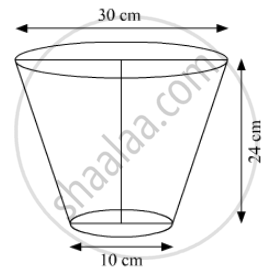Share

# The Diameters of the Lower and Upper Ends of a Bucket in the Form of a Frustum of a Cone Are 10 Cm and 30 Cm Respectively. If Its Height is 24 Cm, Find: - CBSE Class 10 - Mathematics

ConceptSurface Area of a Combination of Solids

#### Question

The diameters of the lower and upper ends of a bucket in the form of a frustum of a cone are 10 cm and 30 cm respectively. If its height is 24 cm, find:

1) The area of the metal sheet used to make the bucket.

2) Why we should avoid the bucket made by ordinary plastic? [Use π = 3.14]

#### SolutionWe have:

A radius of the upper end of the frustum, R = 15 cm; Radius of the lower end of the frustum, r = 5 cm; Height of frustum, = 24 cm

we know

Slant height, l^2 = h^2 + (R - r)^2

=> l^2 = ((24)^2 + (15 - 5)^2) = (576 + 100) = 676

= l = 26 cm

1) Required area of the metal sheet = pi[r^2 + 1(R + r)] sq. cm

= 3.14 [5^2 + 26(15 + 5)] cm^2

= 3.14 xx (25 + 520) cm^2

= 3.14 xx 545 cm^2

= 1711.3 cm^3

2) Plastic is harmful to the environment and to protect the environment its use should be avoided.

Is there an error in this question or solution?

#### Video TutorialsVIEW ALL 

Solution The Diameters of the Lower and Upper Ends of a Bucket in the Form of a Frustum of a Cone Are 10 Cm and 30 Cm Respectively. If Its Height is 24 Cm, Find: Concept: Surface Area of a Combination of Solids.
S